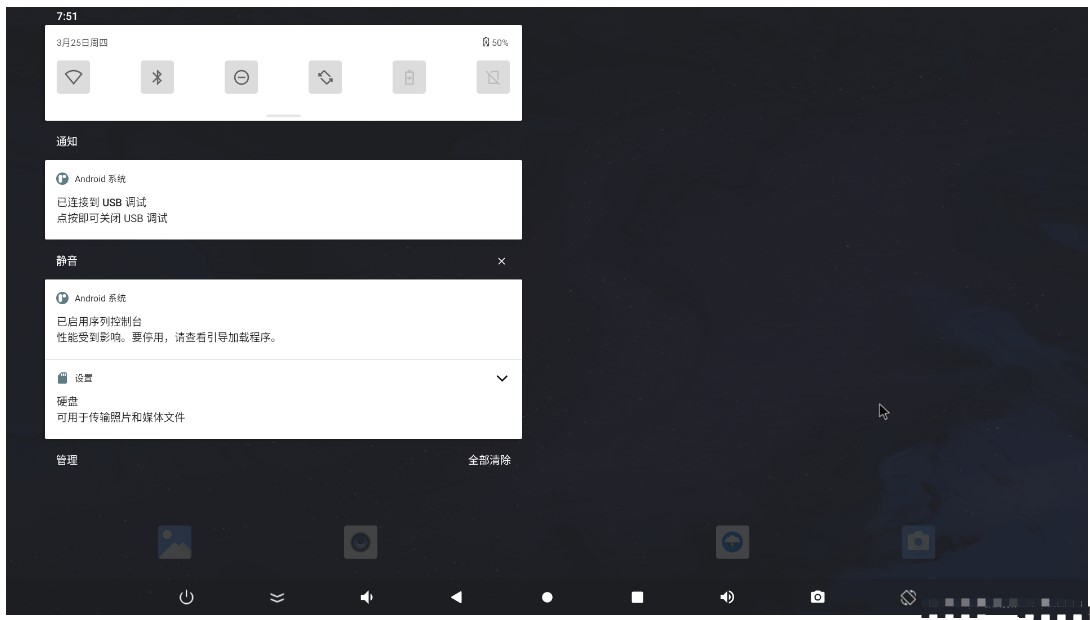• 首页
• 论坛
• 版块

• 问答
• 活动
• 下载
• 视频
• 学院
• 发现

• 工作
• 升级VIP

[资料]

# RK3568 Android11.0 Vold-DiskInfo区分u盘和硬盘

5 天前2473
 .pcb { margin-right: 0 } 1、RK3568 Android11.0 Vold-DiskInfo区分u盘和硬盘 　　测试平台 　　Platform： RK3568 　　OS： Android 11.0 　　需求描述 　　目前系统内部设备没有对硬盘（sata/usb移动硬盘）和u盘做区分，统一显示的为usb存储设备。目前有需求要在显示上有所区分。 　　解决方案 　　如何区分移动硬盘和U盘，见Android usb设备的检测及区分（sata硬盘、优盘及移动硬盘）。主要就是检测removeable值，为0：硬盘，为1:u盘 　　解决补丁如下 　　From 572cf35c07f68c5cb5defe40c536dd9ad1b4648e Mon Sep 17 00:00:00 2001 　　From： sjf 　　Date： Sat， 20 Mar 2021 11:31:51 +0800 　　Subject： ［PATCH］ =？UTF-8？q？Android-》Vold-DiskInfo：=E6=B7=BB=E5=8A=A0？= 　　=？UTF-8？q？=E7=A1=AC=E7=9B=98=E7=B1=BB=E5=9E=8B，=E5=B0=86usb=E5=AD=98？= 　　=？UTF-8？q？=E5=82=A8=E8=AE=BE=E5=A4=87=E5=8C=BA=E5=88=86u=E7=9B=98？= 　　=？UTF-8？q？=E5=92=8C=E7=A1=AC=E7=9B=98（U=E7=9B=98=E6=98=BE=E7=A4=BAusb=20d？= 　　=？UTF-8？q？river，usb/sata=E7=A1=AC=E7=9B=98=E6=98=BE=E7=A4=BAHardDisk）？= 　　MIME-Version： 1.0 　　Content-Type： text/plain; charset=UTF-8 　　Content-Transfer-Encoding： 8bit原作者：风之空响163.13 KB , 下载次数: 0淘帖 显示全部楼层

### 评论

 .kl3w_guisepost { padding: 0px 5px;} .fpd a, .fpd div { float: left;} 您需要登录后才可以回帖 登录 | 注册elecfans短短

## 站长推荐/7•最新主题
•推荐主题
•热门主题
•我的帖子
-

-

-

-

-

-

-

-

-

-

-